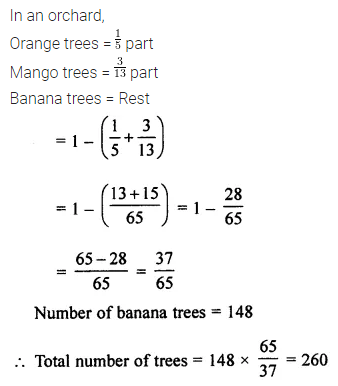## ML Aggarwal Class 7 Solutions for ICSE Maths Chapter 2 Fractions and Decimals Ex 2.3

Question 1.
Evaluate the following: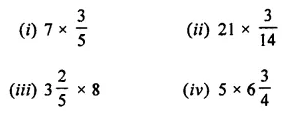Solution:Question 2.
Find: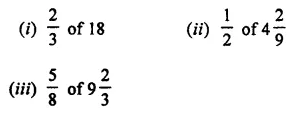Solution: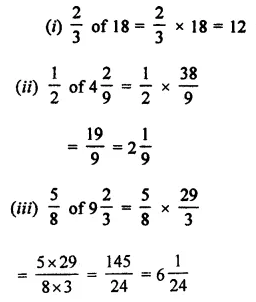Question 3.
Evaluate the following: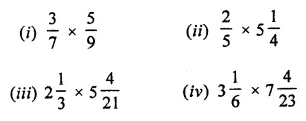Solution: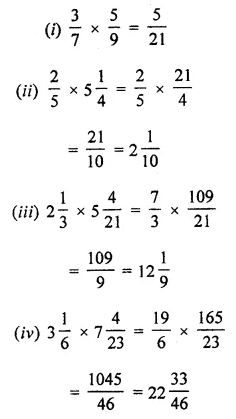Question 4.
Find the value of: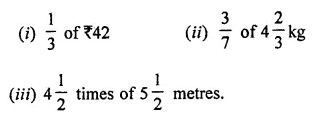Solution:Question 5.
Which is greater: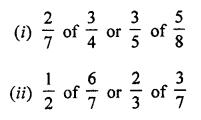Solution: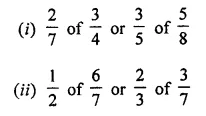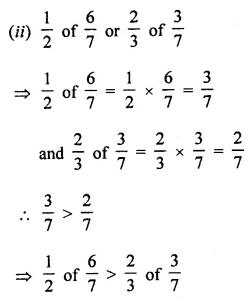Question 6.
If 1 metre cloth costs ₹ 31$$\frac { 3 }{ 4 }$$, find the cost of 5$$\frac { 1 }{ 2 }$$ metres cloth.
Solution: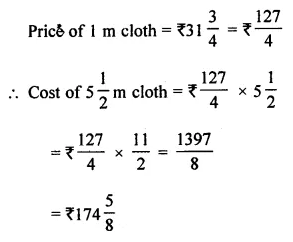Question 7.
If the speed of a car is 105$$\frac { 1 }{ 5 }$$ km/h, find the distance covered by it in 3$$\frac { 3 }{ 5 }$$ hours.
Solution:Question 8.
A car runs 16 km using 1 litre of petrol. How much distance will it cover in 2$$\frac { 3 }{ 4 }$$ litres of petrol?
Solution: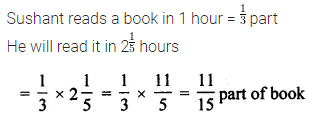Question 9.
Sushant reads $$\frac { 1 }{ 3 }$$ part of a book in 1 hour. How much part of the book will he read in 2$$\frac { 1 }{ 5 }$$ hours?
Solution:Question 10.
An ornament is made of gold and copper and weighs 52 grams. If $$\frac { 2 }{ 13 }$$ of its part is copper, find the weight of pure gold in it.
Solution:Question 11.
In a class of 40 students, $$\frac { 1 }{ 5 }$$ of the total number of students like to study English and $$\frac { 2 }{ 5 }$$ of the total number of students like to study Mathematics and the remaining like to study Science.
(i) How many students like to study English?
(ii) How many students like to study Mathematics?
(iii) What fraction of the total number of students like to study Science?
Solution: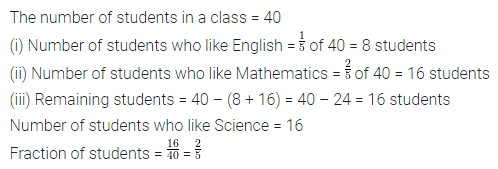Question 12.
A rectangular sheet of paper is 12$$\frac { 1 }{ 2 }$$ cm long and 10$$\frac { 2 }{ 3 }$$ cm wide. Find its
(i) perimeter
(ii) area
Solution:Question 13.
In a school, $$\frac { 25 }{ 54 }$$ of the students are girls and the rest are boys. If the number of boys is 2030, find the number of girls.
Solution: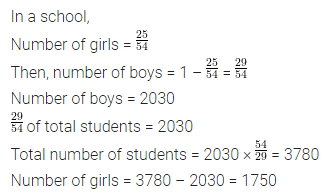Question 14.
In an orchard, $$\frac { 1 }{ 5 }$$ are orange grees, $$\frac { 3 }{ 13 }$$ are mango trees and the rest are banana trees. If the banana trees are 148 in number, find the total number of trees in the orchard.
Solution: